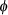# A force of 5 pounds stretches

A force of 5 pounds stretches a spring 1 foot. A mass weighing 6.4 pounds is attached to the spring, and the system is then immersed in a medium that offers a damping force numerically equal to 1.6 times the instantaneous velocity.

I calculated that the equation of motion if the mass is initially released from rest from a point 1 foot above the equilibrium position is : x(t) = e^(-4t) [-cos(3t) – 4/3sin(3t)]

Please help me find the equation of motion in the form x(t) = Ae^(??t) sin(sqrt(?^2 – ?^2) +)

-When i tried getting this i got A =4/3, w^2 = 25, lambda = 16, pheta = arctan(3/4)

Find the first time at which the mass passes through the equilibrium position heading upward

_________________S

Please help me find the equation of motion in the form x(t) = Ae^(??t) sin(sqrt(?^2 – ?^2) +)

x(t) = _____________________